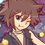# Algebra (Thailand Math POSN 1st elimination round 2014)

Way too easier than last year.

Write the full solution.

1.) Let $$a,b,c,d$$ be real numbers such that $$\displaystyle \frac{a}{b} = \frac{b}{c} = \frac{c}{d} = \frac{d}{a}$$. Find the maximum value of $$\displaystyle \frac{ab-3bc+ca}{a^{2}-b^{2}+c^{2}}$$.

2.) If $(x+y+z)\left(\displaystyle \frac{1}{x}+\frac{1}{y}+\frac{1}{z}\right) = 1$, prove that $(x+y)(y+z)(z+x) = 0$.

3.) Find all roots of $\displaystyle \left\lfloor \frac{x}{2} \right\rfloor - \left\lfloor \frac{x}{3} \right\rfloor = \frac{x}{7}$.

4.) (Someone posted this problem before in Brilliant.) Find all polynomials $P(x)$ with real coefficients such that $P(1) = 210$ and

$(x+10)P(2x) = (8x-32)P(x+6)$.

5.) If $x,y,z$ are positive real numbers such that $\displaystyle x+\frac{y}{z} = y+\frac{z}{x} = z+\frac{x}{y} = 2$. Find the value of $x+y+z$.

Check out all my notes and stuffs for more math problems!

Thailand Math POSN 2013

Thailand Math POSN 2014Note by Samuraiwarm Tsunayoshi
6 years, 11 months ago

This discussion board is a place to discuss our Daily Challenges and the math and science related to those challenges. Explanations are more than just a solution — they should explain the steps and thinking strategies that you used to obtain the solution. Comments should further the discussion of math and science.

When posting on Brilliant:

• Use the emojis to react to an explanation, whether you're congratulating a job well done , or just really confused .
• Ask specific questions about the challenge or the steps in somebody's explanation. Well-posed questions can add a lot to the discussion, but posting "I don't understand!" doesn't help anyone.
• Try to contribute something new to the discussion, whether it is an extension, generalization or other idea related to the challenge.

MarkdownAppears as
*italics* or _italics_ italics
**bold** or __bold__ bold
- bulleted- list
• bulleted
• list
1. numbered2. list
1. numbered
2. list
Note: you must add a full line of space before and after lists for them to show up correctly
paragraph 1paragraph 2

paragraph 1

paragraph 2

[example link](https://brilliant.org)example link
> This is a quote
This is a quote
    # I indented these lines
# 4 spaces, and now they show
# up as a code block.

print "hello world"
# I indented these lines
# 4 spaces, and now they show
# up as a code block.

print "hello world"
MathAppears as
Remember to wrap math in $$ ... $$ or $ ... $ to ensure proper formatting.
2 \times 3 $2 \times 3$
2^{34} $2^{34}$
a_{i-1} $a_{i-1}$
\frac{2}{3} $\frac{2}{3}$
\sqrt{2} $\sqrt{2}$
\sum_{i=1}^3 $\sum_{i=1}^3$
\sin \theta $\sin \theta$
\boxed{123} $\boxed{123}$

Sort by:

- 6 years, 11 months ago

Nope there's another case of a,b,c,d you didn't consider.

- 6 years, 11 months ago

For1st I got 3

- 6 years, 11 months ago

Yep, but how will you prove that no.5 has exactly 1 answer?

- 6 years, 11 months ago

We can apply AM-GM first on $x,y,z$ and then on $\frac{x}{y},\frac{y}{z},\frac{z}{x}$ and add both and say that since it is at its minimum so there is equality between the terms.

- 6 years, 10 months ago